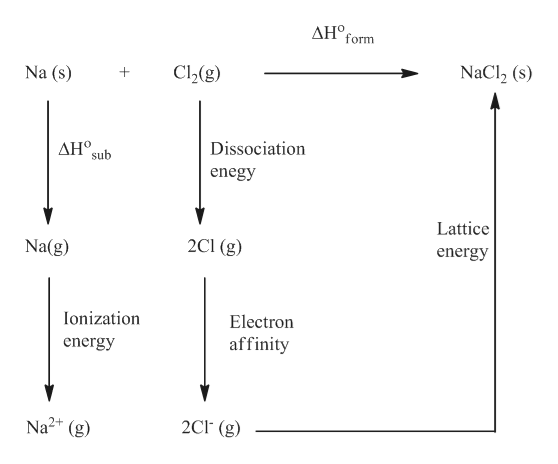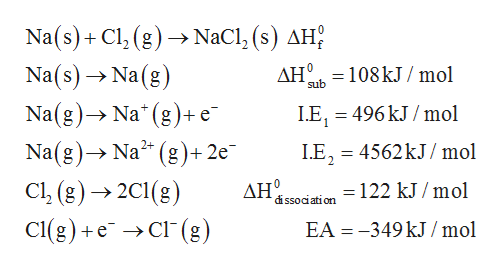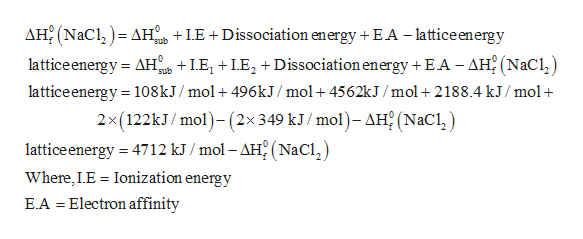# Construct a Born-Haber cycle for the formation of the hypothetical compound NaCl2, where the sodium ion has a 2+ charge (the 2nd ionization energy for sodium is given in Table 7.2 in the textbook).Part AHow large would the lattice energy need to be for the formation of NaCl2 to be exothermic? Part BIf we were to estimate the lattice energy of NaCl2 to be roughly equal to that of MgCl2 (2326 kJ/mol from Table 8.2 in the textbook), what value would you obtain for the standard enthalpy of formation, ΔH∘f, of NaCl2?

Question
109 views

#### Part A

How large would the lattice energy need to be for the formation of NaCl2 to be exothermic?

#### Part B

If we were to estimate the lattice energy of NaCl2 to be roughly equal to that of MgCl2 (2326 kJ/mol from Table 8.2 in the textbook), what value would you obtain for the standard enthalpy of formation, ΔH∘f, of NaCl2?
check_circle

Step 1

The Born-Haber cycle for the formation of hypothetical compound NaCl2 can be constructed as given below.help_outlineImage TranscriptioncloseΔΗ , form Na (s) C2(g) NaCl2 (s) Dissociation ΔΗ. sub enegy Lattice Na(g) 2C1 (g) energy Ionization Electron affinity energy Na2+ (g) 2Cl (g) fullscreen
Step 2

The various reactions are given as,help_outlineImage TranscriptioncloseNa(s)+Cl, (g)NaCl, (s) AH? Na(s)Na (g) Na (g) Na (g)+e AH -108kJ/mol sub LE, 496 kJ/mol Na(g)Na2 Cl, (g) 2C1(g) Cl(g)e C(g) (g+2e LE, 4562kJ/mol -122 kJ /mol dissociation EA -349 kJ /mol fullscreen
Step 3

Using Hess&rsqu...help_outlineImage TranscriptioncloseAH2 (NaC AH LE +Dissociation energy + EA - lattice energy latticeenergy AH LEDissociation energy + EA - AH2 (NaCl lattice energy 108kJ/mol+ 496KJ /mol+4562kJ /mol + 2188.4 kJ/mol 2x(122KJ/ mol)-(2x349 kJ/ mol)- AH (NaCl) sub sub lattice energy 4712 kJ /mol - AH2 (NaCl Where, LE Ionization energy = E.A Electron affinity = fullscreen

### Want to see the full answer?

See Solution

#### Want to see this answer and more?

Solutions are written by subject experts who are available 24/7. Questions are typically answered within 1 hour.*

See Solution
*Response times may vary by subject and question.
Tagged in

### Chemistry